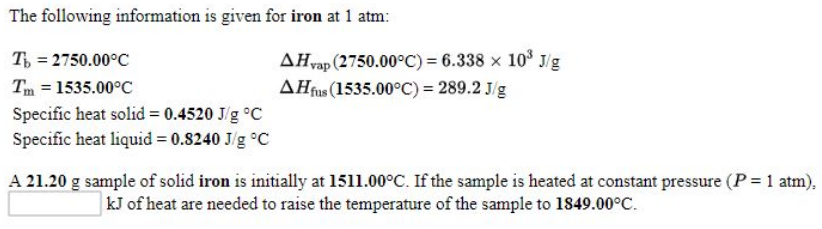# Problem: he following information is given for iron at 1 atm: Tb = 2750.00°C        ΔHvap (2750.00°C) = 6.338 x 103 J/gTm = 1535.00°C        ΔHfus (1535.00°C) = 289.2 J/gSpecific heat solid = 0.4520 J g°C Specific heat liquid = 0.8240 J g°C   A 21.20 g sample of solid iron is initially at 1511.00°C. If the sample is heated at constant pressure (P = 1 atm).________kJ of heat are needed to raise the temperature of the sample to 1849.00°C.

###### FREE Expert Solution###### Problem Details

he following information is given for iron at 1 atm:

Tb = 2750.00°C        ΔHvap (2750.00°C) = 6.338 x 103 J/g

Tm = 1535.00°C        ΔHfus (1535.00°C) = 289.2 J/g

Specific heat solid = 0.4520 J g°C

Specific heat liquid = 0.8240 J g°C

A 21.20 g sample of solid iron is initially at 1511.00°C. If the sample is heated at constant pressure (P = 1 atm).

________kJ of heat are needed to raise the temperature of the sample to 1849.00°C.